## 3.5Matrix construction

The construction of each matrix equation involves building the matrix and source coeﬃcients from the terms in the equation being solved, with further adjustments for boundary conditions.

The ﬁgure below illustrates the process of building a matrix equation for a ﬁeld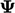from an equation including advection, diﬀusion and source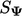.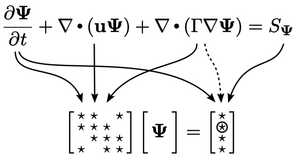Coeﬃcients for matrix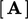and source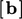are calculated for each individual term in the equation, e.g.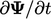,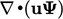, etc. using the discretisation methods described in this chapter.

The overall coeﬃcients are calculated as the sum of coeﬃcients for each term in the the equation. Most terms contribute to both the matrix and source coeﬃcients, although this depends on the choice of discretisation scheme.

Finally, boundary conditions are incorporated into the equation through further adjustments to coeﬃcients in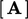and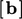as shown below. The adjustments, principally from the advection and diﬀusion terms, are applied to coeﬃcients corresponding to cells at the domain boundary.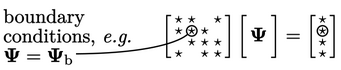### Implicit and explicit

The equation for a ﬁeld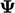on the previous page is discretised to form the matrix equation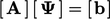. The discretisation of a term is implicit when it contributes to coeﬃcients in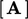by treatingas the solved ﬁeld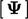.

Explicit discretisation calculates coeﬃcients inonly, using current values of ﬁelds. When solving an equation for, derivatives without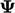must be explicit. Terms withcould be treated explicitly by using current values of, but they generally are not, since explicit solutions are unstable beyond a limiting time step as described in Sec. 3.17 . A notable exception to this are the terms discussed in Sec. 3.20 .

The curl derivative, e.g.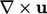, includes terms in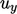and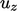in the decoupled matrix equation for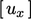. These terms must be treated explicitly since they do not include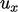itself. The situation for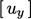and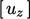is the same, so the curl derivative can be explicit only.

This leaves the following terms which are generally treated implicitly:3

• time derivative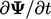;
• diﬀusion (Laplacian)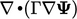;
• advection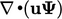;
• implicit linear function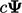, where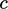is a scalar.

This chapter details: the discretisation of these terms, which are generally treated implicitly; and, other terms such as divergence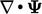and gradient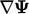which can only be discretised explicitly within a segregated solution.

3also equivalent terms including density, e.g.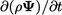,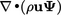Notes on CFD: General Principles - 3.5 Matrix construction